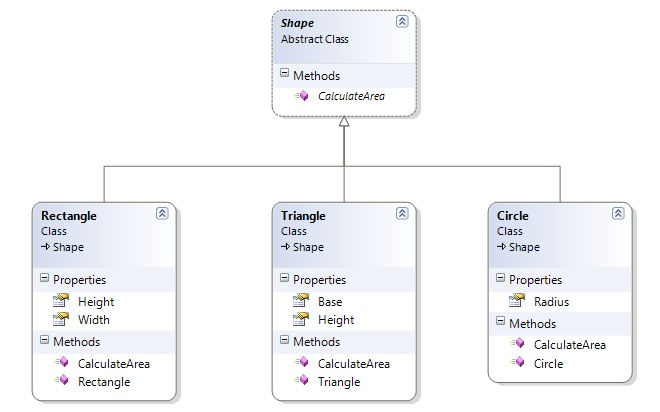# Open Close Principle With C#

Open Close Principle is an Object Oriented Design principle. It is first introduced by Betrand Meyer in 1988. He says “Software entities (Class, module, function etc.) should be open for extension, but closed for modification”. An entity is “Open for extension” means that its behavior can be extended to accommodate new demand. The entity is “closed for modification” means that the existing source code of the module is not changed or minimum change when making enhancement. It is clear that if a system cannot accommodate change easily, its life cycle will end fast.

Sometimes code changes introduce heavy risk. At the time of changing, you must ensure that changes will not break the system. Sometimes it takes huge regression testing. This risk can be minimized if no changes are made to existing code.

So, our intention should be writing code in such a way that new functionality should be added with minimum changes or not changes in the existing code. It should be done in a way to allow the adding of new functionality as new classes, keeping as much as possible existing code unchanged. The major advantages of “open close principle” is that it undergo changes and its value will be tremendous. It required almost no regression testing.

Let’s introduce open close principle with an example. Suppose, in our application, we need a “Area calculator” which calculate area of rectangle. However, in this occasion we just create a class AreaCalculator then there will be a method RectangleArea in this class which just calculates area of rectangle. It works fine. In the middle of the application development, we need to calculate area of Triangle and Circle. In this occasion, what should we do? We just add another two method TriangleArea and CircleArea and can do the job. But several problems will arise here – for each new shape you have to add new unit of code. Developer must have to know the logic to calculate area of new shape. Adding a new shape might effect in existing functionalities. So, it will take huge cost of regression testing. This is actually violate, open close principle.

We implement the same problem abide by open close principle by the following way. Here Rectangle, Triangle and Circle class inherit the Shape class and implement CalculateArea Method. In this way, if you need to calculate area of x shape just add a class of x and then implement shape and calculate area of x without modifying exiting code.

Class diagram:Open Close Principle Implementation by C#:

Step 1: Create abstract Shape class

``````using System;
using System.Collections.Generic;
using System.Linq;
using System.Text;

namespace OCP
{
public abstract class Shape
{
public abstract double CalculateArea();
}
}
``````

Step 2: Create Rectangle class

``````using System;
using System.Collections.Generic;
using System.Linq;
using System.Text;

namespace OCP
{
public class Rectangle : Shape
{
public double Height { get; set; }
public double Width { get; set; }

public Rectangle(double height, double width)
{
this.Height = height;
this.Width = width;
}

public override double CalculateArea()
{
return Height * Width;
}
}
}

``````

Step 3: Create Triangle class

``````using System;
using System.Collections.Generic;
using System.Linq;
using System.Text;

namespace OCP
{
public class Triangle : Shape
{
public double Base { get; set; }
public double Height { get; set; }

public Triangle(double vbase, double vheight)
{
this.Base = vbase;
this.Height = vheight;
}

public override double CalculateArea()
{
return 1 / 2.0 * Base * Height;
}
}
}

``````

Step 4: Create circle class

``````using System;
using System.Collections.Generic;
using System.Linq;
using System.Text;

namespace OCP
{
public class Circle : Shape
{
public double Radius { get; set; }

{
}

public override double CalculateArea()
{
}
}
}

``````

Step 5: Client class which uses Rectangle, Triangle and Circle class

``````using System;
using System.Collections.Generic;
using System.Linq;
using System.Text;

namespace OCP
{
public class Program
{
public static void Main(string[] args)
{
Shape objShape = new Rectangle(20, 30);
Console.WriteLine("Area of Rectangle: " + objShape.CalculateArea());

objShape = new Triangle(20, 30);
Console.WriteLine("Area of Triangle: " + objShape.CalculateArea());

objShape = new Circle(4);
Console.WriteLine("Area of Circle: " + objShape.CalculateArea());